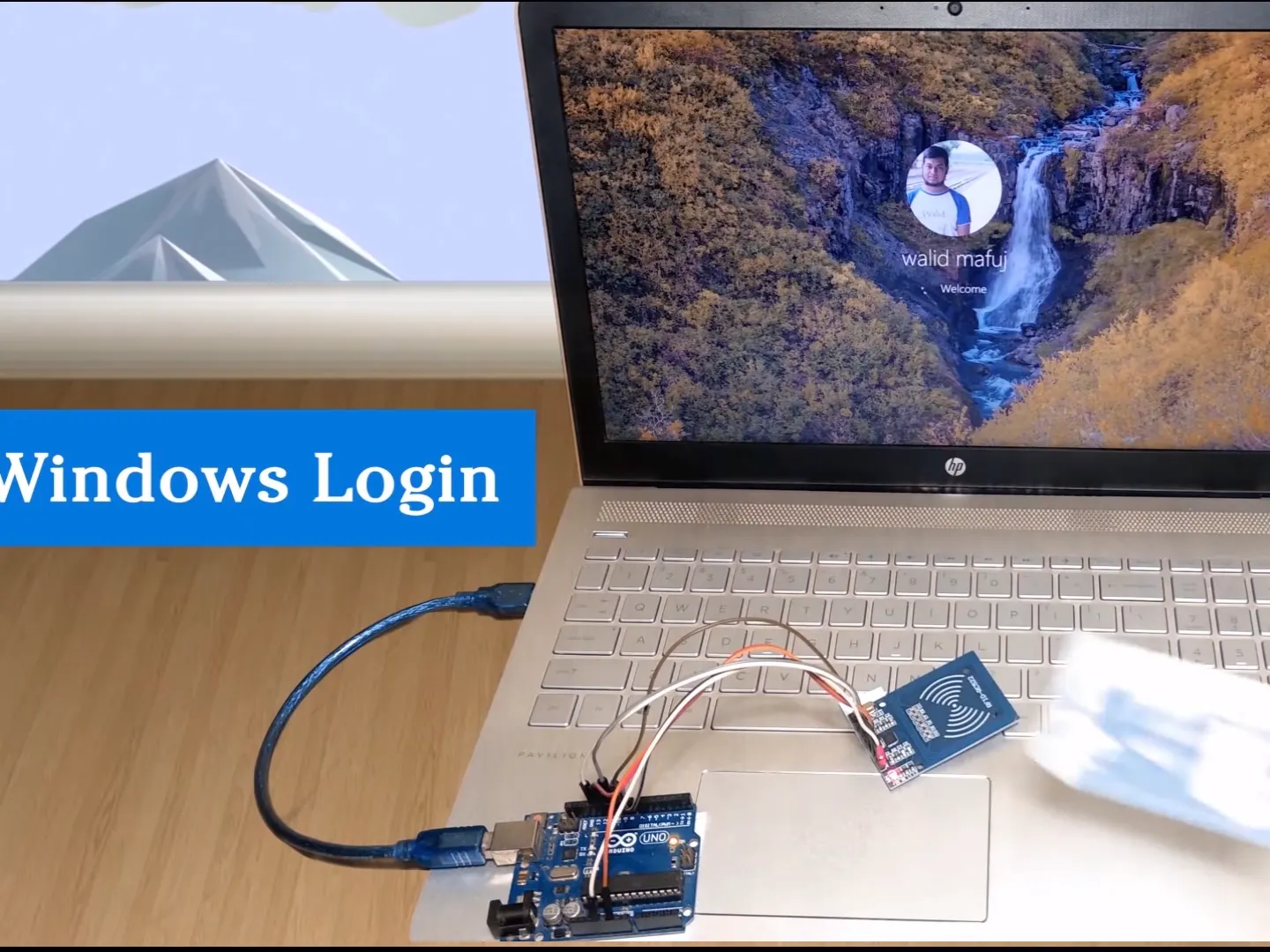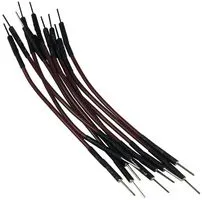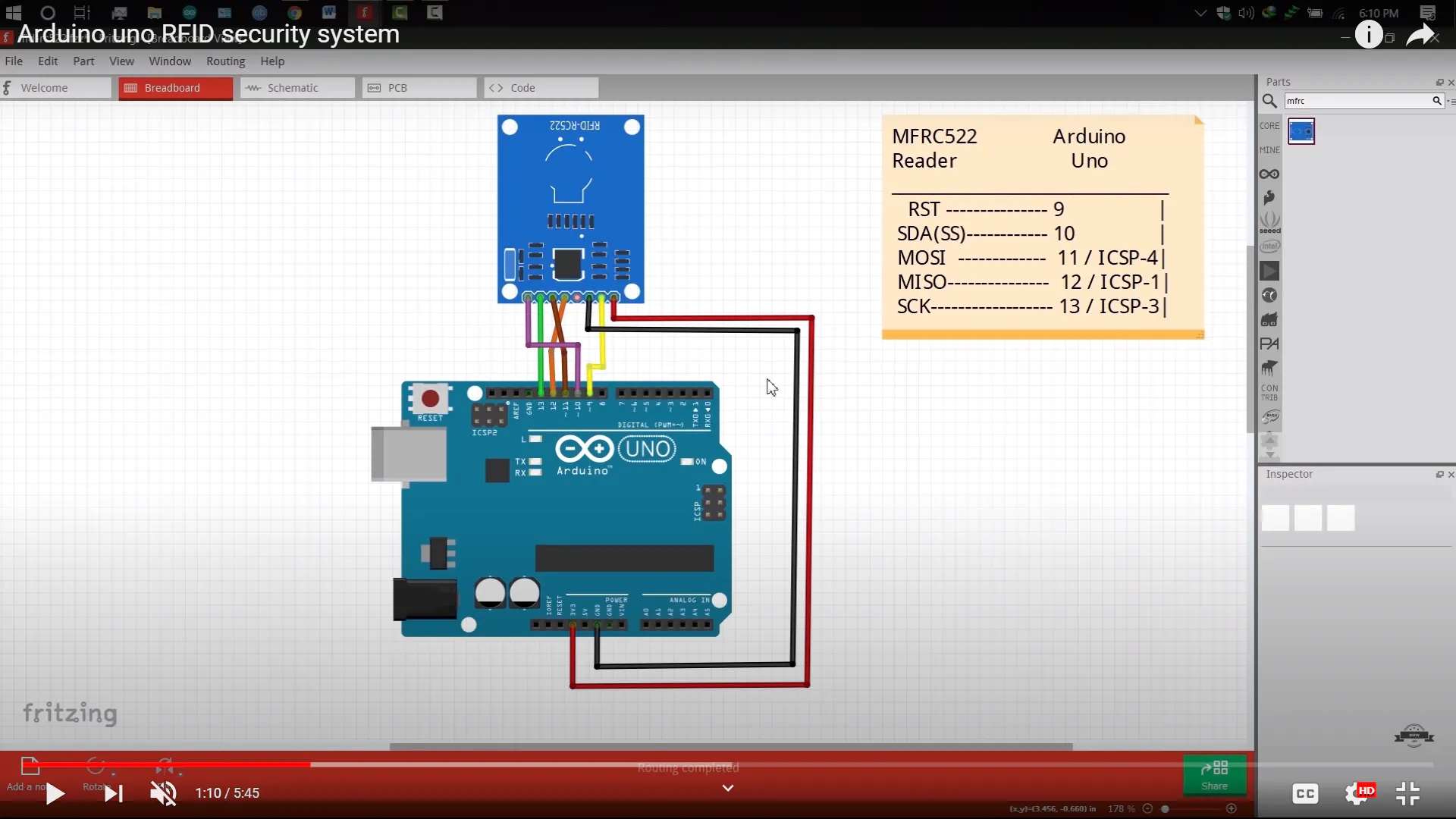# Arduino uno RFID security system for PC / laptop## Things used in this project

### Hardware componentsArduino UNO
×1
 MFRC522
×1

### Software apps and online servicesArduino IDE
 Flip software
 UnoJoy

### Hand tools and fabrication machines10 Pc. Jumper Wire Kit, 5 cm Long

## Schematics

### MFRC522## Code

Arduino
```//=====================================Walid Mafuj===================================//
uint8_t buf = { 0 };
#include <SPI.h>
#include <MFRC522.h> // RFID library
#define SS_PIN 10 //RX slave select
#define RST_PIN 9
int gled = 7; // optional
int rled = 4;// optional
MFRC522 mfrc522(SS_PIN, RST_PIN); // Create MFRC522 instance.
String card_ID=""; //
String rfid="2222123818";// UID (unique Id Code Of Your Rfid Tag)
void setup() {
Serial.begin(9600); // Initialize serial communications with the PC
SPI.begin();  // Init SPI bus
mfrc522.PCD_Init(); // Init MFRC522 card
pinMode(gled,OUTPUT);
pinMode(rled,OUTPUT);
}
void loop() {
//look for new card
if ( ! mfrc522.PICC_IsNewCardPresent()) {
return;
}
return;//if read card serial(0) returns 1, the uid struct contians the ID of the read card.
}
for (byte i = 0; i < mfrc522.uid.size; i++) {
card_ID += mfrc522.uid.uidByte[i];
}
// Serial.println(card_ID);
if(card_ID==rfid){digitalWrite(gled,HIGH);
pressKey("enter"); releaseKey("enter");
digitalWrite(gled,LOW); delay(200);digitalWrite(gled,HIGH); delay(200);digitalWrite(gled,LOW);
}
digitalWrite(rled,HIGH);    digitalWrite(rled,LOW); delay(200);digitalWrite(rled,HIGH); delay(200);digitalWrite(rled,LOW);  }      else{ goto cont;}
delay(1000);
cont:
delay(1000);
card_ID="";
}
boolean isModifier(int keycode) {
boolean result = false;
if (keycode >= 224 && keycode <= 231) { // if the keycode is a modifier key
result = true;
}
return result;
}

void pressModifier(String keyname) {
pressModifier(getKeycode(keyname));
}
void pressModifier(int keycode) {
if (isModifier(keycode)) { // if the keycode represents a modifier key
Serial.write(buf, 8); // Send key report
}
}

void releaseModifier(String keyname) {
releaseModifier(getKeycode(keyname));
}
void releaseModifier(int keycode) {
if (isModifier(keycode)) { // if the keycode represents a modifier key
Serial.write(buf, 8); // Send key report
}
}
void releaseAllModifiers() {
buf = B00000000;
Serial.write(buf, 8); // Send key report
}

void pressKey(String keyname) {
pressKey(getKeycode(keyname));
}
void pressKey(int keycode) { // TODO: cycle the 6 key spots in the report buffer instead of just using buf each time.
buf = keycode;
Serial.write(buf, 8); // Send key report
}

void releaseKey(String keyname) {
releaseKey(getKeycode(keyname));
}
void releaseKey(int keycode) {
// find the keycode in the report buffer, then set it to zero.
int i=0;
for (i=2; i<8; i++) {
if (buf[i] == keycode) {
buf[i] = 0;
}
}
Serial.write(buf, 8); // Send key report
}
void releaseAllKeys() {
int i=0;
for (i=2; i<8; i++) {
buf[i] = 0;
}
Serial.write(buf, 8); // Send key report
}

void pressSequenceOfKeys(const char * keySequence[], int numberOfKeys) {
// This function can be good for pressing a few keys while holding a modifier down for example.

int i = 0;
for (i=0; i<numberOfKeys; i++) {
pressKey(keySequence[i]);
releaseKey(keySequence[i]);
}
}

void typeLiteralString(String string) {
char charArray[string.length()+1];
string.toCharArray(charArray, string.length()+1);
typeLiteralString(charArray, string.length());
}
void typeLiteralString(char string[], int stringLength) { // stringLength is the length of the printable string without considering the null byte.
// This function will type the given string exactly as given, automatically pressing left_shift where necessary for capitals and symbols.

// just in case:
releaseAllKeys();
releaseAllModifiers();

boolean charNeedsShift = false;
boolean shiftIsPressed = false;

int i=0;
for (i=0; i<stringLength; i++) {
charNeedsShift = characterNeedsShift(string[i]);
if (charNeedsShift && !shiftIsPressed) {
pressModifier("left_shift");
shiftIsPressed = true;
}
else if (!charNeedsShift && shiftIsPressed) {
releaseModifier("left_shift");
shiftIsPressed = false;
}
pressKey(String(string[i])); // without converting the char in string[i] to a String, arduino would prefer the pressKey(int) function instead of the pressKey(String) function, casting the char to a keycode (int) instead of a keyname (String).
releaseKey(String(string[i])); // same as previous comment, but with releaseKey().
}

releaseAllModifiers();
}

boolean characterNeedsShift(char character) {
int needsModifier = false;
if ( // look up an ascii table and this will make sense.
(character >= 33 && character <= 38)
|| (character >= 40 && character <= 43)
|| (character == 58)
|| (character == 60)
|| (character >= 62 && character <= 90)
|| (character >= 94 && character <= 95)
|| (character >= 123 && character <= 126)
) {
needsModifier = true;
}
return needsModifier;
}

int getKeycode(String keyname) {
String key = String(keyname); // Use a copy so that we don't mutate the user's String. Not sure if this is needed, but just in case. TODO: find out.
key.toLowerCase();

int keycode = 0; // keycode of zero means nothing pressed.

// non-modifier keys
if      (key == "a") { keycode =  4; }
else if (key == "b") { keycode =  5; }
else if (key == "c") { keycode =  6; }
else if (key == "d") { keycode =  7; }
else if (key == "e") { keycode =  8; }
else if (key == "f") { keycode =  9; }
else if (key == "g") { keycode = 10; }
else if (key == "h") { keycode = 11; }
else if (key == "i") { keycode = 12; }
else if (key == "j") { keycode = 13; }
else if (key == "k") { keycode = 14; }
else if (key == "l") { keycode = 15; }
else if (key == "m") { keycode = 16; }
else if (key == "n") { keycode = 17; }
else if (key == "o") { keycode = 18; }
else if (key == "p") { keycode = 19; }
else if (key == "q") { keycode = 20; }
else if (key == "r") { keycode = 21; }
else if (key == "s") { keycode = 22; }
else if (key == "t") { keycode = 23; }
else if (key == "u") { keycode = 24; }
else if (key == "v") { keycode = 25; }
else if (key == "w") { keycode = 26; }
else if (key == "x") { keycode = 27; }
else if (key == "y") { keycode = 28; }
else if (key == "z") { keycode = 29; }

else if (key == "1" || key == "!") { keycode = 30; }
else if (key == "2" || key == "@") { keycode = 31; }
else if (key == "3" || key == "#") { keycode = 32; }
else if (key == "4" || key == "\$") { keycode = 33; }
else if (key == "5" || key == "%") { keycode = 34; }
else if (key == "6" || key == "^") { keycode = 35; }
else if (key == "7" || key == "&") { keycode = 36; }
else if (key == "8" || key == "*") { keycode = 37; }
else if (key == "9" || key == "(") { keycode = 38; }
else if (key == "0" || key == ")") { keycode = 39; }

else if (key == "enter" || key == "return")     { keycode = 40; }
else if (key == "escape" || key == "")    { keycode = 41; }
else if (key == "backspace" || key == "") { keycode = 42; }
else if (key == "tab" || key == "  ")      { keycode = 43; }
else if (key == "space" || key == " ")      { keycode = 44; }

else if (key == "-" || key == "_")  { keycode = 45; }
else if (key == "=" || key == "+")  { keycode = 46; }
else if (key == "[" || key == "{")  { keycode = 47; }
else if (key == "]" || key == "}")  { keycode = 48; }
else if (key == "\\" || key == "|") { keycode = 49; }
else if (key == ";" || key == ":")  { keycode = 51; }
else if (key == "'" || key == "\"") { keycode = 52; }
else if (key == "`" || key == "~")  { keycode = 53; }
else if (key == "," || key == "<")  { keycode = 54; }
else if (key == "." || key == ">")  { keycode = 55; }
else if (key == "/" || key == "?")  { keycode = 56; }

// TODO: Fix these keycodes. V
else if (key == "capslock")         { keycode = 58; }

else if (key == "f1")      { keycode = 59; }
else if (key == "f2")      { keycode = 60; }
else if (key == "f3")      { keycode = 61; }
else if (key == "f4")      { keycode = 62; }
else if (key == "f5")      { keycode = 63; }
else if (key == "f6")      { keycode = 64; }
else if (key == "f7")      { keycode = 65; }
else if (key == "f8")      { keycode = 66; }
else if (key == "f9")      { keycode = 67; }
else if (key == "f10")     { keycode = 68; }
else if (key == "f11")     { keycode = 69; }
else if (key == "f12")     { keycode = 70; }

else if (key == "print_screen")    { keycode = 70; }
else if (key == "scroll_lock")     { keycode = 71; }
else if (key == "pause")           { keycode = 72; }
else if (key == "insert")          { keycode = 73; }
else if (key == "home")            { keycode = 74; }
else if (key == "page_up")         { keycode = 75; }
else if (key == "delete")          { keycode = 76; }
else if (key == "end")             { keycode = 77; }
else if (key == "page_down")       { keycode = 78; }

else if (key == "right_arrow")     { keycode = 79; }
else if (key == "left_arrow")      { keycode = 80; }
else if (key == "down_arrow")      { keycode = 81; }
else if (key == "up_arrow")        { keycode = 82; }

else if (key == "numlock" || key == "clear")   { keycode = 83; }

//TODO: keypad and miscellaneous keys if you want them.

// modifier keys.
else if (key == "left_control")  { keycode = 224; }
else if (key == "left_shift")    { keycode = 225; }
else if (key == "left_alt")      { keycode = 226; }
else if (key == "left_gui")      { keycode = 227; }

else if (key == "right_control") { keycode = 228; }
else if (key == "right_shift")   { keycode = 229; }
else if (key == "right_alt")     { keycode = 230; }
else if (key == "right_gui")     { keycode = 231; }

return keycode;
}

}
int getModifierMask(int keycode) { // return value of 0 means key is not a modifier.

// NOTE: these are not the usage keycodes like for other keys, but rather the bit masks.
if      (keycode == 224) { modifiermask = B00000001; } // left ctrl
else if (keycode == 225) { modifiermask = B00000010; } // left shift
else if (keycode == 226) { modifiermask = B00000100; } // left alt
else if (keycode == 227) { modifiermask = B00001000; } // left gui
else if (keycode == 228) { modifiermask = B00010000; } // right ctrl
else if (keycode == 229) { modifiermask = B00100000; } // right shift
else if (keycode == 230) { modifiermask = B01000000; } // right alt
else if (keycode == 231) { modifiermask = B10000000; } // right gui